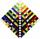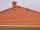Difference AP

Calculate the difference of arithmetic progression if the sum of its first 19 members Sn = 8075 and the first member is a1 = 20

Result

d =  45

Solution:Leave us a comment of example and its solution (i.e. if it is still somewhat unclear...):Be the first to comment!Next similar examples:

1. Difference AP 4Calculate the difference of the AP if a1 = 0.5, a2 + a3 = -1.1
2. AS sequenceIn an arithmetic sequence is given the difference d = -3 and a71 = 455. a) Determine the value of a62 b) Determine the sum of 71 members.
3. AP - basicsDetermine first member and differentiate of the the following sequence: a3-a5=24 a4-2a5=61
4. Sequence 3Write the first 5 members of an arithmetic sequence: a4=-35, a11=-105.
5. Sum of membersWhat is the sum of the first two members of the aritmetic progression if d = -4.3 and a3 = 7.5?
6. SequenceWrite the first 6 members of these sequence: a1 = 5 a2 = 7 an+2 = an+1 +2 an
7. Sequence 2Write the first 5 members of an arithmetic sequence a11=-14, d=-1
8. SequenceWrite the first 7 members of an arithmetic sequence: a1=-3, d=6.
9. SeatsSeats in the sport hall are organized so that each subsequent row has five more seats. First has 10 seats. How many seats are: a) in the eighth row b) in the eighteenth row
10. AP - simpleDetermine the first nine elements of sequence if a10 = -1 and d = 4
11. SequenceBetween numbers 1 and 53 insert n members of the arithmetic sequence that its sum is 702.
12. Third memberDetermine the third member of the AP if a4=93, d=7.5.
13. SeriesYour task is express the sum of the following arithmetic series for n = 14: S(n) = 11 + 13 + 15 + 17 + ... + 2n+9 + 2n+11
14. RoofTiles are stacked in rows on the trapezoidal shaped roof. At the ridge is 15 tiles and each subsequent row has one more tile than in the previous row. How many tiled is covered roof if lowermost row has 37 tiles?
15. GP membersThe geometric sequence has 10 members. The last two members are 2 and -1. Which member is -1/16?
16. Theorem proveWe want to prove the sentence: If the natural number n is divisible by six, then n is divisible by three. From what assumption we started?
17. Parametric equationFind the parametric equation of a line with y-intercept (0,-4) and a slope of -2.